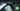# Hackerrank | Solution of Apple and Orange in Golang

September 19th, 2018
|

In this post we will solve question apple and orange from hackerrank implementation section. A very easy one, let's dive right into it.

# Problem Statement

The question can be found at this link. The problem statement states that we are given two trees, an apple and an orange tree, and fruits are falling from those trees. We need to find out if those fruits are falling in a given line segment or not.

# Challenges

• Choose our preferred language as golang on hackerrank. The moment we do that, we get some 50-60 lines of code which are very unfamiliar to someone who is new to language.
• Cracking the logic required to solve the question.

# Solution

This is the template you get on hackerrank for this problem statement.

``````    package main

import (
"bufio"
"fmt"
"io"
"os"
"strconv"
"strings"
)

// Complete the countApplesAndOranges function below.
func countApplesAndOranges(s int32, t int32, a int32, b int32, apples []int32, oranges []int32) {

}

func main() {

sTemp, err := strconv.ParseInt(st, 10, 64)
checkError(err)
s := int32(sTemp)

tTemp, err := strconv.ParseInt(st, 10, 64)
checkError(err)
t := int32(tTemp)

aTemp, err := strconv.ParseInt(ab, 10, 64)
checkError(err)
a := int32(aTemp)

bTemp, err := strconv.ParseInt(ab, 10, 64)
checkError(err)
b := int32(bTemp)

mTemp, err := strconv.ParseInt(mn, 10, 64)
checkError(err)
m := int32(mTemp)

nTemp, err := strconv.ParseInt(mn, 10, 64)
checkError(err)
n := int32(nTemp)

var apples []int32

for i := 0; i < int(m); i++ {
applesItemTemp, err := strconv.ParseInt(applesTemp[i], 10, 64)
checkError(err)
applesItem := int32(applesItemTemp)
apples = append(apples, applesItem)
}

var oranges []int32

for i := 0; i < int(n); i++ {
orangesItemTemp, err := strconv.ParseInt(orangesTemp[i], 10, 64)
checkError(err)
orangesItem := int32(orangesItemTemp)
oranges = append(oranges, orangesItem)
}

countApplesAndOranges(s, t, a, b, apples, oranges)
}

if err == io.EOF {
return ""
}

return strings.TrimRight(string(str), "\r\n")
}

func checkError(err error) {
if err != nil {
panic(err)
}
}``````
• First go ahead and delete everything in the pre-existing template.
• Let's write down our main wrapper function.
``````package main
import "fmt"

func main(){

}``````

Let's figure out the variables we need for this problem. We need to store the co-ordinates of line segment `s,t`, we need co-ordinates of the apple tree `a` and the co-ordinates of the orange tree `b`. We need the number of apples falling `m`, the number of oranges falling `n`. Now do we need to store the entire array or can we just have a temporary variable, store input one by one, decide if it falls under the line segment and be done with it? we will go with second approach. So we need a temporary variable `temp`. Next we need two counter variables to store the number of times an apple or orange falls in the given segment, so two more variables, `ac and oc`. Phew!!! let's declare them and take the required inputs.

``````    ...
var s,t,a,b,m,n,temp,ac,oc int
fmt.Scan(&s,&t)
fmt.Scan(&a,&b)
fmt.Scan(&m,&n)``````

Okay, now we have our variables, next we will take the distance that a fruit travels after falling as input one by one and calculate if it lies inside the line segment. Now if the co-ordinate of the tree when added to the distance lies inside the segment we will increase the counter else no increase, and same logic for orange tree as well. It doesn't matter if the distance is negative or positive, the summation will always provide desired result. So here goes the code.

``````	...
for i:= 0;i<m;i++{
fmt.Scan(&temp)
if a+temp >= s && a+temp <= t {
ac++
}
}
for i:= 0;i<n;i++{
fmt.Scan(&temp)
if b+temp >= s && b+temp <= t {
oc++
}
}
fmt.Printf("%d\n%d",ac,oc)``````

So we have two loops here, completely similar, we'll just discuss one. We are looping the number of fruits time, taking an input in the temporary variable, now if the co-ordinate of apple or orange tree plus the distance the fruit travels is greater than equal to `s` and less than equal to `t` we say that the fruit fell inn the segment and increase the occurence of that event. After the loops we just print the out, since we are required to print the output in two lines, we use formatted printing, `%d` for integer, `\n` for new line. Now the complete code should look like

``````package main

import "fmt"

func main(){
var s,t,a,b,m,n,temp,ac,oc int
fmt.Scan(&s,&t)
fmt.Scan(&a,&b)
fmt.Scan(&m,&n)

for i:= 0;i<m;i++{
fmt.Scan(&temp)
if a+temp >= s && a+temp <= t {
ac++
}
}
for i:= 0;i<n;i++{
fmt.Scan(&temp)
if b+temp >= s && b+temp <= t {
oc++
}
}
fmt.Printf("%d\n%d",ac,oc)
}``````

Let's review what are the highlights to take away from this question. We take a bunch of variables as input and then discussed the logic. Next we take the distance each fruit travels as input one by one and add it to tree co-ordinate to check if it falls in the line segment, and finally print our result in the desired format. This is it for this one, complete source code for this post can be found on my Github Repo. Will see you in the next one.